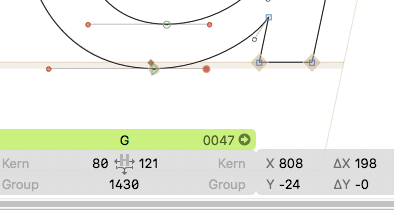# Problem with fractional coordinates

Hi all,

After doing some transformations on a glyph in Python the resulting coordinates are fractional. Even though I set the grid spacing back to 1 afterwards and round all the coordinates in a script, the nodes are still coloured red in Glyphs as they are floating point numbers that differ from the integers after 10 decimal places or so:

``````# round all coordinates
for path in Layer.paths:
for node in path.nodes:
node.x = int(round(node.x))
node.y = int(round(node.y))
if (node.x != round(node.x)):
print ("fractional coordinates in ", node, node.x, round(node.x))
``````

results in:

``````fractional coordinates in  <GSNode 0x60016401bae0> 610 -24 CURVE SMOOTH 609.9999999999998 610
``````Is there a way I can set the coordinates back to the integer values they were before the transformation?

I looked but couldn’t find a method to remove fractional coordinates in one go.

Best,
Tamir

1 Like

Those differences are to be expected with floating points. Direct comparison is not going to work. So you need to do:

``````if abs(node.x - round(node.x)) > 0.0001:
``````

And the plugin that draws the red dots probably need to do the same.

But when you set the coordinates and the font has a grid, it should round the numbers automatically.

1 Like

Before processing, the node coordinates look like this, i.e.:

``````print("current grid", Glyphs.font.gridMain())

for path in Layer.paths:
for node in path.nodes:
print (node, node.x, node.y)
``````

results in:

``````current grid 1
<GSNode 0x6000224bedc0> 808 -24 OFFCURVE 808.0 -24.0
...
``````

After processing (setting grid to zero, rotating by the slant angle and then rotating back by the same angle), it looks like this, i.e.:

``````# round all coordinates
for path in Layer.paths:
for node in path.nodes:
node.x = int(round(node.x))
node.y = int(round(node.y))

Glyphs.font.setGridMain_( 1 )

print("after")

for path in Layer.paths:
for node in path.nodes:
node.x = int(round(node.x))
node.y = int(round(node.y))
print (node, node.x, node.y)
``````

results in:

``````after
<GSNode 0x6000224bedc0> 808 -24 OFFCURVE 807.9999999999999 -24.0
``````

So what is the difference between the node before processing and afterwards? How are they stored internally? Are both floating-point numbers? If so, why is one equal to exactly 808.0 and the other isn’t? This seems to be what’s causing the plugin to paint the node red. Can I restore the previous state programmatically?

Just to make it clear, it doesn’t seem to make a difference if I round the nodes before or after setting the grid to 1; in fact, in this example, I do both. Even moving the path by hand by 1 unit and back doesn’t round the handles.

Best,
Tamir

Can you send me a full script that I can run this myself?

I’ve sent you the filter to the support email. Thanks!